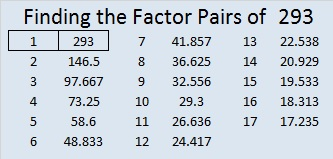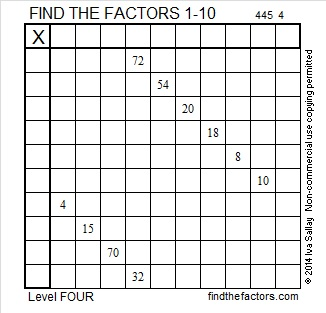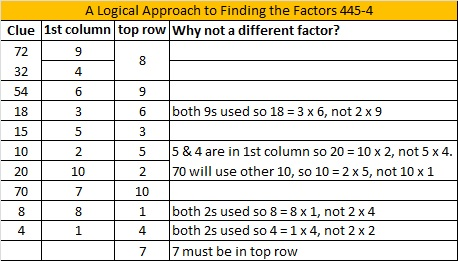# 293 and Level 4

• 293 is a prime number.
• Prime factorization: 293 is prime.
• The exponent of prime number 293 is 1. Adding 1 to that exponent we get (1 + 1) = 2. Therefore 293 has exactly 2 factors.
• Factors of 293: 1, 293
• Factor pairs: 293 = 1 x 293
• 293 has no square factors that allow its square root to be simplified. √293 ≈ 17.117How do we know that 293 is a prime number? If 293 were not a prime number, then it would be divisible by at least one prime number less than or equal to √293 ≈ 17.117. Since 293 cannot be divided evenly by 2, 3, 5, 7, 11, 13, or 17, we know that 293 is a prime number.

—————

I will be having major surgery tomorrow so I’ll publish the rest of this week’s puzzles early. I suspect that next week’s puzzles might be published late.

Today’s puzzle has another ten clues, but this puzzle is just a little more challenging that the last one.Print the puzzles or type the factors on this excel file: 10 Factors 2014-11-10

Here are a few more number facts about the number 293:

17² + 2² = 293

Thus, 293 is the hypotenuse of a primitive Pythagorean triple:

• 68-285-293 calculated from 2(17)(2), 17² – 2², 17² + 2².

293 is the short leg of this primitive Pythagorean triple:

• 293-42924-42925

293 can also be written as the sum of three squares four different ways:

• 16² + 6² + 1² = 293
• 15² + 8² + 2² = 293
• 14² + 9² + 4² = 293
• 12² + 10² + 7² = 293## 2 thoughts on “293 and Level 4”

1.Paula Beardell Krieg

Good Luck with your surgery! I will be counting the days until you are back.

2.wbhs62

Very best wishes for a speedy recovery.

This site uses Akismet to reduce spam. Learn how your comment data is processed.